# 基础算法

#### 枚举

1.输出1~5的所有排列

2.输出1~n的所有子集的子集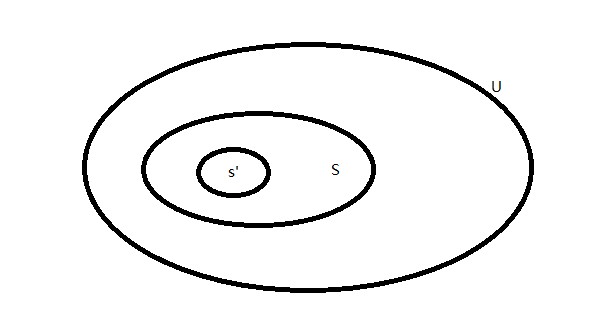U是全集，S是子集，s'是子集的子集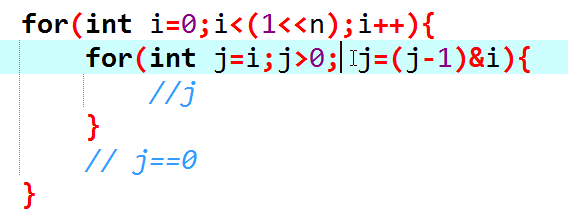#### 搜索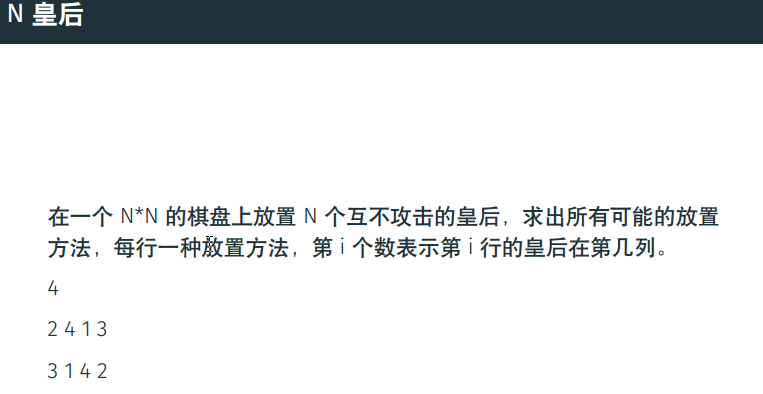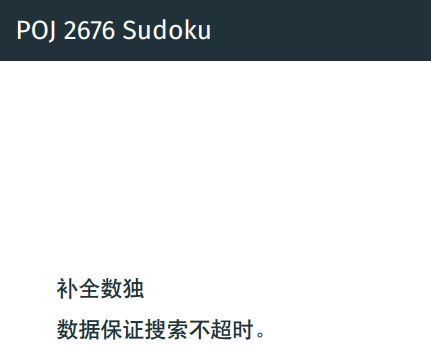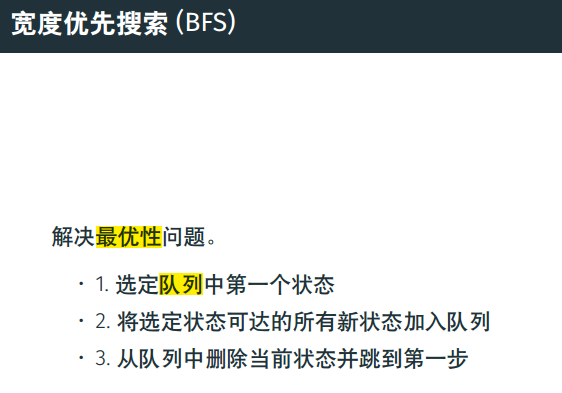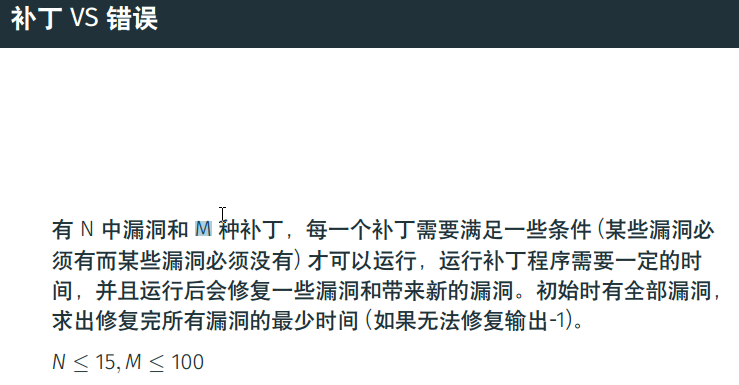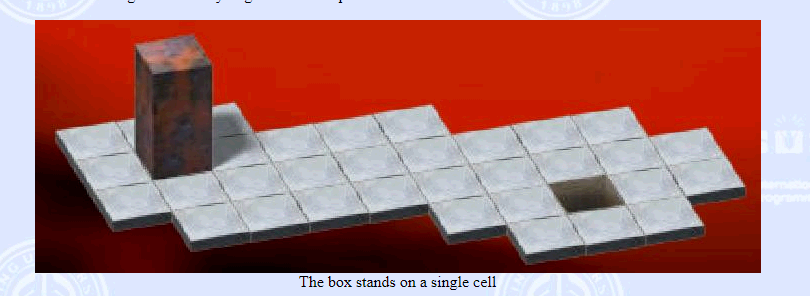#include<cstdio>
#include<algorithm>

using namespace std;
int n,m;
int ans;
int small;
int small_q;
void dfs(int x,int v,int q, int R,int H){
// 已经考虑完成最下面x层
// 第x层 R,H
// 当前蛋糕体积为v
if(v>n) return;

if(x==m){
if(v==n){
ans = min(ans,q);
}
return ;
}
if(v + small[m-x] > n) return;
if(q + small_q[m-x] >= ans) return;
int big = v;
for(int i=1;i<=m-x;i++){
big += (R-i)*(R-i)*(H-i);
}
if(big<n) return;
// x+1
for(int i=m-x;i<R;i++){
for(int j=m-x;j<H;j++){
dfs(x+1, i*i*j+v, 2*i*j+q,i,j);
}
}
}
int main(){
http://poj.org/problem?id=1011&lang=zh-CN
ans = 1e9;
scanf("%d%d",&n,&m);
for(int i=1;i<=20;i++){
small[i] = small[i-1] + i*i*i;
small_q[i] = small_q[i-1]+2*i*i;
}
// 最底一层
for(int i=1;i<=n;i++){
if(i*i>n) break;
for(int j=1;j<=n;j++){
dfs(1,i*i*j, 2*i*j + i*i,i,j);
}
}
if(ans == int(1e9)) puts("0");
else{
printf("%d\n",ans);
}
return 0;
}
##### 扩展

$A^*,IDA^*$

#### 分治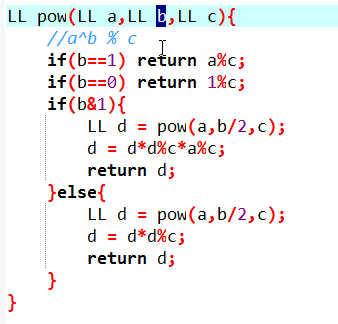ex:快(gui)速乘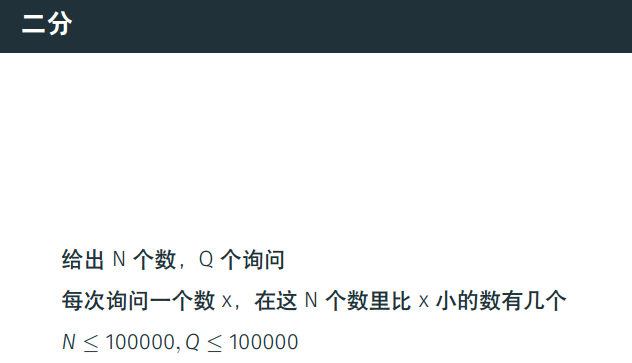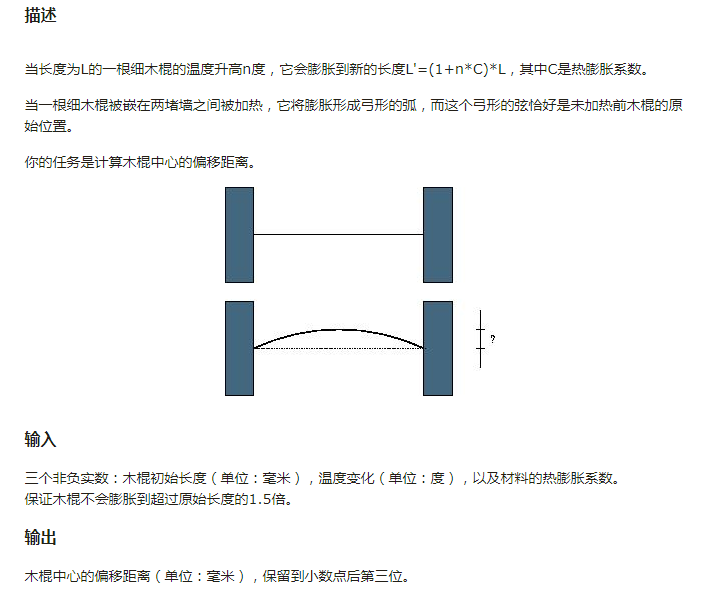#### 贪心

$a_i\geq d_i$时，肯定按$d_i$从小到大打

$a_i<d_i$时，怎么打呢？

1.按$d-a$从小到大

2.按$d$从小到大

3.按$d$从大到小

4.按$a$从小到大

5.按$a$从大到小

5为什么是对的？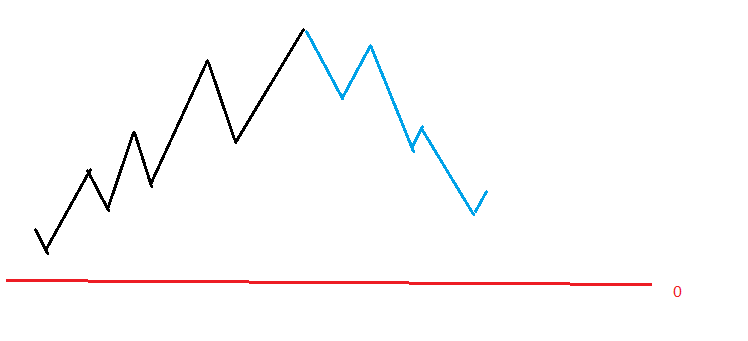$d-a$:血量:100 $d_1=98,a_1=96,d_2=96,a_2=94$

$d$从小到大：同上

$d$从大到小：血量：100,$d_1=98,a_1=1,d_2=97,a_2=96$

$a$从小到大：显然反了

#### 数组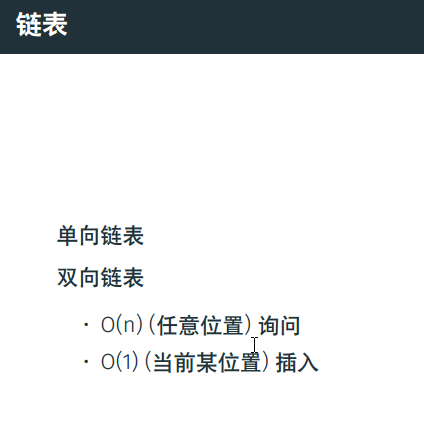#### 单调栈&单调队列poj P2559

###### 单调队列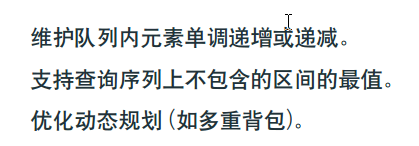#### RMQ问题

###### ST表

ST表要处理一些区间，来使这些区间可以覆盖任意一个区间。

$st[i][j]=max\{[i][j-1],st[i+(1<<(j-1))][j-1]\}$。在覆盖的时候，我们可以算出$log_2{len}$来知道$k$。由于$c++$自带的函数太慢了，所以我们进行预处理。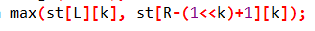###### 二维ST表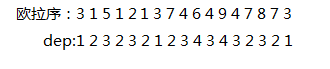#### 并查集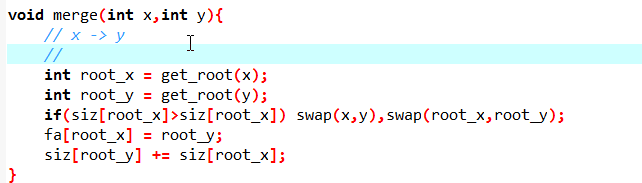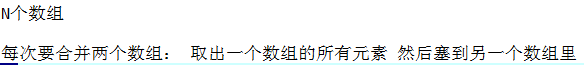posted @ 2020-01-18 18:00  千载煜  阅读(...)  评论(...编辑  收藏